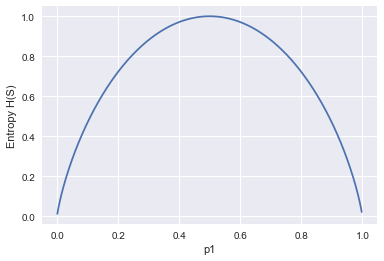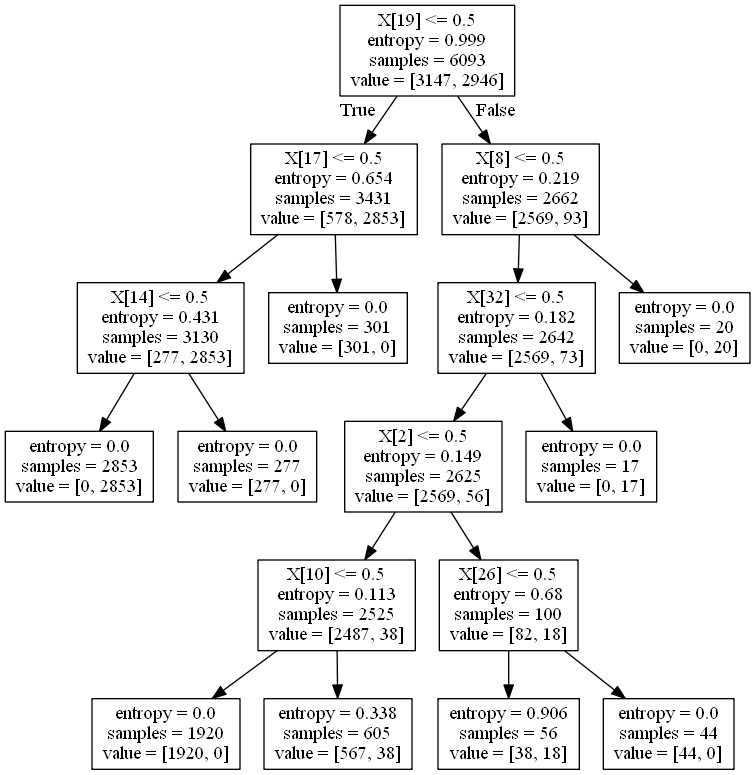In :
# 決定木

# モジュール
import numpy as np
import scipy as sp
import pandas as pd
import sklearn

import matplotlib.pyplot as plt
import matplotlib as mpl
import seaborn as sns
sns.set()
%matplotlib inline

%precision 3

import requests
import zipfile
import io

In :
# サンプルデータを取得
url = 'https://archive.ics.uci.edu/ml/machine-learning-databases/mushroom/agaricus-lepiota.data'
res = requests.get(url).content

In :
# デコードして まともに読めるようにする

Out:
0 1 2 3 4 5 6 7 8 9 ... 13 14 15 16 17 18 19 20 21 22
0 p x s n t p f c n k ... s w w p w o p k s u
1 e x s y t a f c b k ... s w w p w o p n n g
2 e b s w t l f c b n ... s w w p w o p n n m
3 p x y w t p f c n n ... s w w p w o p k s u
4 e x s g f n f w b k ... s w w p w o e n a g

5 rows × 23 columns

In :
# ラベルの確認
url = 'https://archive.ics.uci.edu/ml/machine-learning-databases/mushroom/agaricus-lepiota.names'
res = requests.get(url).content
res = res.decode('utf-8')
print(res,sep='\n')

1. Title: Mushroom Database

2. Sources:
(a) Mushroom records drawn from The Audubon Society Field Guide to North
American Mushrooms (1981). G. H. Lincoff (Pres.), New York: Alfred
A. Knopf
(b) Donor: Jeff Schlimmer (Jeffrey.Schlimmer@a.gp.cs.cmu.edu)
(c) Date: 27 April 1987

3. Past Usage:
1. Schlimmer,J.S. (1987). Concept Acquisition Through Representational
Adjustment (Technical Report 87-19).  Doctoral disseration, Department
of Information and Computer Science, University of California, Irvine.
--- STAGGER: asymptoted to 95% classification accuracy after reviewing
1000 instances.
2. Iba,W., Wogulis,J., & Langley,P. (1988).  Trading off Simplicity
and Coverage in Incremental Concept Learning. In Proceedings of
the 5th International Conference on Machine Learning, 73-79.
Ann Arbor, Michigan: Morgan Kaufmann.
-- approximately the same results with their HILLARY algorithm
3. In the following references a set of rules (given below) were
learned for this data set which may serve as a point of
comparison for other researchers.

Duch W, Adamczak R, Grabczewski K (1996) Extraction of logical rules
from training data using backpropagation networks, in: Proc. of the
The 1st Online Workshop on Soft Computing, 19-30.Aug.1996, pp. 25-30,
available on-line at: http://www.bioele.nuee.nagoya-u.ac.jp/wsc1/

Duch W, Adamczak R, Grabczewski K, Ishikawa M, Ueda H, Extraction of
crisp logical rules using constrained backpropagation networks -
comparison of two new approaches, in: Proc. of the European Symposium
on Artificial Neural Networks (ESANN'97), Bruge, Belgium 16-18.4.1997,
pp. xx-xx

Wlodzislaw Duch, Department of Computer Methods, Nicholas Copernicus
University, 87-100 Torun, Grudziadzka 5, Poland
e-mail: duch@phys.uni.torun.pl
WWW     http://www.phys.uni.torun.pl/kmk/

Date: Mon, 17 Feb 1997 13:47:40 +0100
From: Wlodzislaw Duch <duch@phys.uni.torun.pl>
Organization: Dept. of Computer Methods, UMK

I have attached a file containing logical rules for mushrooms.
It should be helpful for other people since only in the last year I
have seen about 10 papers analyzing this dataset and obtaining quite
complex rules. We will try to contribute other results later.

With best regards, Wlodek Duch
________________________________________________________________

Logical rules for the mushroom data sets.

Logical rules given below seem to be the simplest possible for the
mushroom dataset and therefore should be treated as benchmark results.

Disjunctive rules for poisonous mushrooms, from most general
to most specific:

P_1) odor=NOT(almond.OR.anise.OR.none)
120 poisonous cases missed, 98.52% accuracy

P_2) spore-print-color=green
48 cases missed, 99.41% accuracy

P_3) odor=none.AND.stalk-surface-below-ring=scaly.AND.
(stalk-color-above-ring=NOT.brown)
8 cases missed, 99.90% accuracy

P_4) habitat=leaves.AND.cap-color=white
100% accuracy

Rule P_4) may also be

P_4') population=clustered.AND.cap_color=white

These rule involve 6 attributes (out of 22). Rules for edible
mushrooms are obtained as negation of the rules given above, for
example the rule:

odor=(almond.OR.anise.OR.none).AND.spore-print-color=NOT.green

gives 48 errors, or 99.41% accuracy on the whole dataset.

Several slightly more complex variations on these rules exist,
involving other attributes, such as gill_size, gill_spacing,
stalk_surface_above_ring, but the rules given above are the simplest
we have found.

4. Relevant Information:
This data set includes descriptions of hypothetical samples
corresponding to 23 species of gilled mushrooms in the Agaricus and
Lepiota Family (pp. 500-525).  Each species is identified as
definitely edible, definitely poisonous, or of unknown edibility and
not recommended.  This latter class was combined with the poisonous
one.  The Guide clearly states that there is no simple rule for
determining the edibility of a mushroom; no rule like leaflets
three, let it be'' for Poisonous Oak and Ivy.

5. Number of Instances: 8124

6. Number of Attributes: 22 (all nominally valued)

7. Attribute Information: (classes: edible=e, poisonous=p)
1. cap-shape:                bell=b,conical=c,convex=x,flat=f,
knobbed=k,sunken=s
2. cap-surface:              fibrous=f,grooves=g,scaly=y,smooth=s
3. cap-color:                brown=n,buff=b,cinnamon=c,gray=g,green=r,
pink=p,purple=u,red=e,white=w,yellow=y
4. bruises?:                 bruises=t,no=f
5. odor:                     almond=a,anise=l,creosote=c,fishy=y,foul=f,
musty=m,none=n,pungent=p,spicy=s
6. gill-attachment:          attached=a,descending=d,free=f,notched=n
7. gill-spacing:             close=c,crowded=w,distant=d
9. gill-color:               black=k,brown=n,buff=b,chocolate=h,gray=g,
green=r,orange=o,pink=p,purple=u,red=e,
white=w,yellow=y
10. stalk-shape:              enlarging=e,tapering=t
11. stalk-root:               bulbous=b,club=c,cup=u,equal=e,
rhizomorphs=z,rooted=r,missing=?
12. stalk-surface-above-ring: fibrous=f,scaly=y,silky=k,smooth=s
13. stalk-surface-below-ring: fibrous=f,scaly=y,silky=k,smooth=s
14. stalk-color-above-ring:   brown=n,buff=b,cinnamon=c,gray=g,orange=o,
pink=p,red=e,white=w,yellow=y
15. stalk-color-below-ring:   brown=n,buff=b,cinnamon=c,gray=g,orange=o,
pink=p,red=e,white=w,yellow=y
16. veil-type:                partial=p,universal=u
17. veil-color:               brown=n,orange=o,white=w,yellow=y
18. ring-number:              none=n,one=o,two=t
19. ring-type:                cobwebby=c,evanescent=e,flaring=f,large=l,
none=n,pendant=p,sheathing=s,zone=z
20. spore-print-color:        black=k,brown=n,buff=b,chocolate=h,green=r,
orange=o,purple=u,white=w,yellow=y
21. population:               abundant=a,clustered=c,numerous=n,
scattered=s,several=v,solitary=y
urban=u,waste=w,woods=d

8. Missing Attribute Values: 2480 of them (denoted by "?"), all for
attribute #11.

9. Class Distribution:
--    edible: 4208 (51.8%)
-- poisonous: 3916 (48.2%)
--     total: 8124 instances


In :
# ラベル設定
"""

"""
data.columns = ['classes', 'cap-shape', 'cap-surface', 'cap-color', 'bruises?', 'odor',
'gill-attachment', 'gill-spacing', 'gill-size', 'gill-color', 'stalk-shape',
'stalk-root', 'stalk-surface-above-ring', 'stalk-surface-below-ring',
'stalk-color-above-ring', 'stalk-color-below-ring', 'veil-type', 'veil-color',
'ring-number', 'ring-type', 'spore-print-color', 'population', 'habitat']


Out:
classes cap-shape cap-surface cap-color bruises? odor gill-attachment gill-spacing gill-size gill-color ... stalk-surface-below-ring stalk-color-above-ring stalk-color-below-ring veil-type veil-color ring-number ring-type spore-print-color population habitat
0 p x s n t p f c n k ... s w w p w o p k s u
1 e x s y t a f c b k ... s w w p w o p n n g
2 e b s w t l f c b n ... s w w p w o p n n m
3 p x y w t p f c n n ... s w w p w o p k s u
4 e x s g f n f w b k ... s w w p w o e n a g

5 rows × 23 columns

In :
# 目的変数:classes

# データの形 .shape
data.shape # (8124, 23)

# 欠損値の数 を確認する
data.isnull().sum().sum() # 0　問題なし

Out:
0
In :
# 決定木では　目的変数　説明変数は数値でないとだめ。
"""
カテゴリ変数をダミー変数にする。on-hot エンコーディングという。

"""
data_dummy = pd.get_dummies(data[['gill-color', 'gill-attachment', 'odor', 'cap-color']])

Out:
gill-color_b gill-color_e gill-color_g gill-color_h gill-color_k gill-color_n gill-color_o gill-color_p gill-color_r gill-color_u ... cap-color_b cap-color_c cap-color_e cap-color_g cap-color_n cap-color_p cap-color_r cap-color_u cap-color_w cap-color_y
0 0 0 0 0 1 0 0 0 0 0 ... 0 0 0 0 1 0 0 0 0 0
1 0 0 0 0 1 0 0 0 0 0 ... 0 0 0 0 0 0 0 0 0 1
2 0 0 0 0 0 1 0 0 0 0 ... 0 0 0 0 0 0 0 0 1 0
3 0 0 0 0 0 1 0 0 0 0 ... 0 0 0 0 0 0 0 0 1 0
4 0 0 0 0 1 0 0 0 0 0 ... 0 0 0 1 0 0 0 0 0 0

5 rows × 33 columns

In :
# 目的変数もフラグ化しておく　0 , 1
data_dummy['flg'] = data['classes'].map(lambda x: 1 if x == 'p' else 0)

Out:
0    1
1    0
2    0
3    1
4    0
Name: flg, dtype: int64
In :
# エントロピー　不純度impurityの指標

# クロス集計
# 頭の色がcかどうかで分類できるかやってみる。
data_dummy.groupby(['cap-color_c','flg'])['flg'].count().unstack()

Out:
flg 0 1
cap-color_c
0 4176 3904
1 32 12
In :
"""
cap-colorがc(cap-color_c=1)のとき

→これでは分類できないことがわかった。
"""

Out:
'\ncap-colorがc(cap-color_c=1)のとき\n毒でないのは32 毒なのは12\n→これでは分類できないことがわかった。\n'
In :
# では　gill-color_b　ではどうか？
data_dummy.groupby(['gill-color_b','flg'])['flg'].count().unstack()

Out:
flg 0 1
gill-color_b
0 4208.0 2188.0
1 NaN 1728.0
In :
"""
gill-colorがbとき

"""

Out:
'\ngill-colorがbとき\n毒でないがゼロ\u3000毒であるが1728なので\n識別能力の高い分類ができそう（不純度が低い）\n素晴らしい\n'
In :
#どこで分けるべきかはエントロピーで判断する
"""

エントロピーH(S)　として算出する

S:データの集合
n:カテゴリーの数
p:各カテゴリーに属するデータサンプルの割合

H(S) = -Σ (pi*log2(pi))

"""

# 毒キノコも普通のきのこも半分づつ（0.5）入っている場合のエントロピーH
# これが　乱雑さが最大になっているとき
H = -(0.5 * np.log2(0.5) + 0.5* np.log2(0.5))
print('エントロピー H(S) : ' , H)

エントロピー H(S) :  1.0

In :
# 不純度が低い場合

p1 = 0.001
p2 = 1 - p1
H=0

for pi in [p1 , p2]:
H -= pi * np.log2(pi)

print('エントロピー H(S) : ' , H)

エントロピー H(S) :  0.011407757737461138

In :
# p1 と　エントロピーの関係
from numpy import log2

def calc_entropy(p1):
p2 = p1
H = - p1 * log2(p1) - (1 - p1) * log2(1 - p1)
return H

p1 = np.arange(0.001, 0.999, 0.001)
plt.plot(p1, calc_entropy(p1))
plt.xlabel('p1')
plt.ylabel('Entropy H(S)')

Out:
Text(0,0.5,'Entropy H(S)')In :
# ではダミーデータに話を戻しましょう
print(data_dummy.groupby('flg')['flg'].count())

"""

"""
# 毒キノコでない4208 の割合 0.517971442639094
p1 = data_dummy.groupby('flg')['flg'].count() / data_dummy.groupby('flg')['flg'].count().sum()

# 毒キノコでない3916 の割合 0.48202855736090594
p2 = data_dummy.groupby('flg')['flg'].count() / data_dummy.groupby('flg')['flg'].count().sum()

flg
0    4208
1    3916
Name: flg, dtype: int64

Out:
0.48202855736090594
In :
# 初期エントロピーを算出する
entropy_init = - p1 * log2(p1) - p2 * log2(p2)
print(entropy_init)
"""

"""

0.9990678968724603

In :
# 情報利得 infomation gain
# どの説明変数で分割すればエントロピーの減少を大きくできるかを探る
print(data_dummy.groupby(['cap-color_c', 'flg'])['flg'].count().unstack())

# cap-cplor_cがcでないとき(0)
p1 = 4176 / (4176+3904) # 毒キノコでない
p2 = 1 - p1             # 毒キノコである
entropy_c0 = - p1 * log2(p1) - p2 * log2(p2)
print(entropy_c0)

# cap-cplor_cがcのとき(1)

p1 = 32 / (32+12)  # 毒キノコでない
p2 = 1 - p1        # 毒キノコである
entropy_c1 = - p1 * log2(p1) - p2 * log2(p2)
print(entropy_c1)

flg             0     1
cap-color_c
0            4176  3904
1              32    12
0.9991823984904757
0.8453509366224364

In :
# 分割後の情報利得を計算する
# データ分割後の平均エントロピー

entropy_after = (4176 + 3904) / 8124 * entropy_c0 + (32 + 12) / 8124 * entropy_c1
print(entropy_after)
"""
あまりエントロピーは減少していない
"""

0.9983492394158581

Out:
'\nあまりエントロピーは減少していない\n'
In :
# 情報利得 infomation gain
entropy_init - entropy_after

Out:
0.0007186574566022674
In :
# 決定木モデルの構築
from sklearn.tree import DecisionTreeClassifier
from sklearn.model_selection import train_test_split

# データ分割
X = data_dummy.drop('flg', axis=1)
y = data_dummy['flg']

X_train, X_test, y_train, y_test = train_test_split(X, y, random_state=0)

In :
# 訓練と検証
model = DecisionTreeClassifier(criterion='entropy', max_depth=5,  random_state=0)
model.fit(X_train, y_train)

Out:
DecisionTreeClassifier(class_weight=None, criterion='entropy', max_depth=5,
max_features=None, max_leaf_nodes=None,
min_impurity_decrease=0.0, min_impurity_split=None,
min_samples_leaf=1, min_samples_split=2,
min_weight_fraction_leaf=0.0, presort=False, random_state=0,
splitter='best')
In :
# 結果
print(model.score(X_train, y_train))
print(model.score(X_test, y_test))

0.9908091252256688
0.9921221073362876

In :
# 可視化
from sklearn import tree
import pydotplus

from sklearn.externals.six import StringIO
from IPython.display import Image

dot_data =StringIO()
tree.export_graphviz(model, out_file=dot_data)
graph = pydotplus.graph_from_dot_data(dot_data.getvalue())
Image(graph.create_png())

Out:In [ ]:
# ==== script end====